# How does work Soil hygrometer sensor with an Arduino Nano board?

## Soil hygrometer sensor with an ArduinoHello guys, Welcome back to another article from the SriTu Hobby blog. Today we are going to talk about the Soil Moisture Sensor. Let us first learn what is a soil moisture sensor. We can mainly use it to measure soil moisture. Also, the irrigation system can be easily designed using this sensor. Through it, the moisture in the soil can be monitored and the water system can be properly controlled. This sensor consists of two main parts. We identify the two parts separately.

### The Probe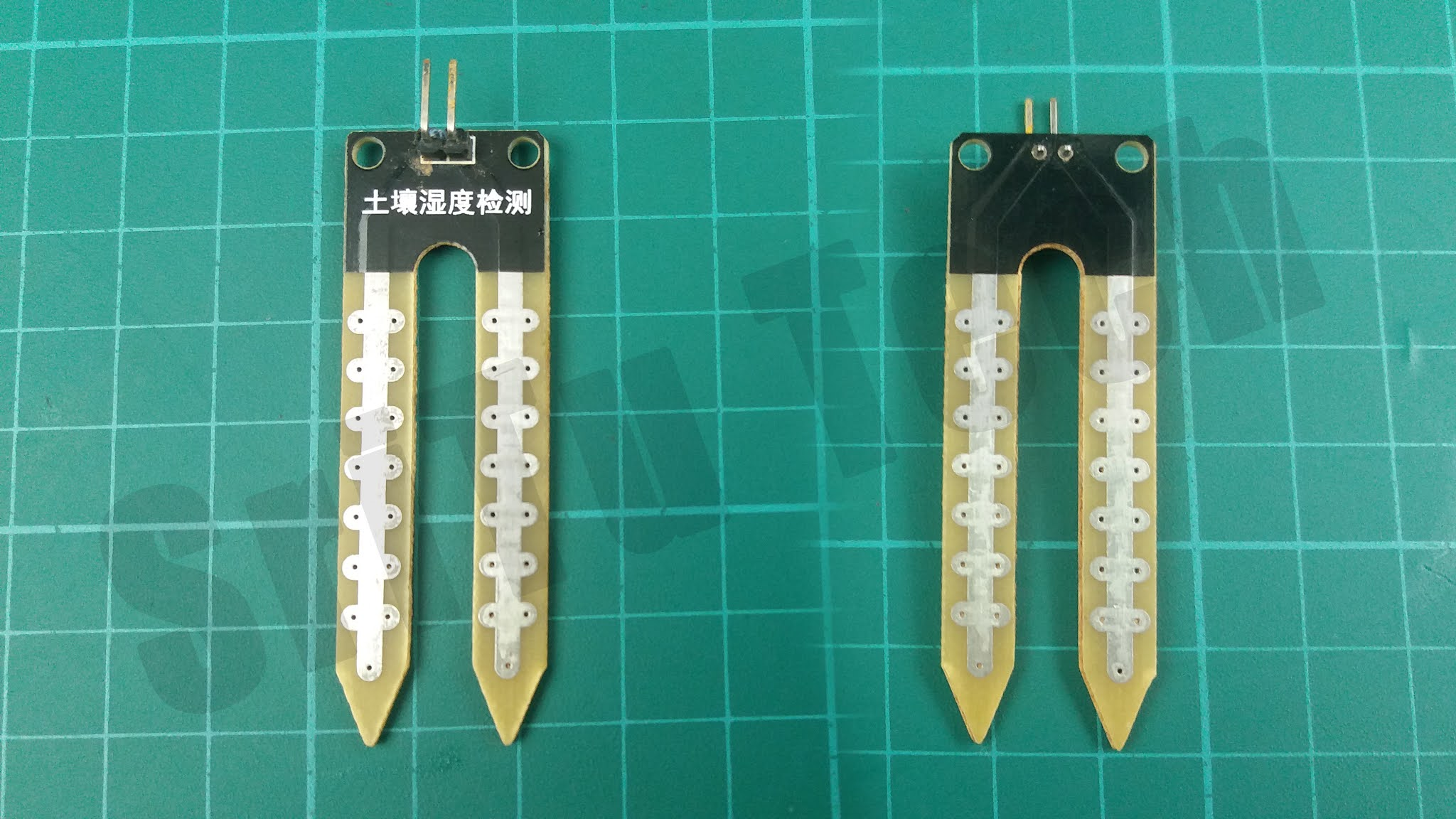This is in the form of a fork and should be inserted into the soil. We will learn later how the probe works.

### The Module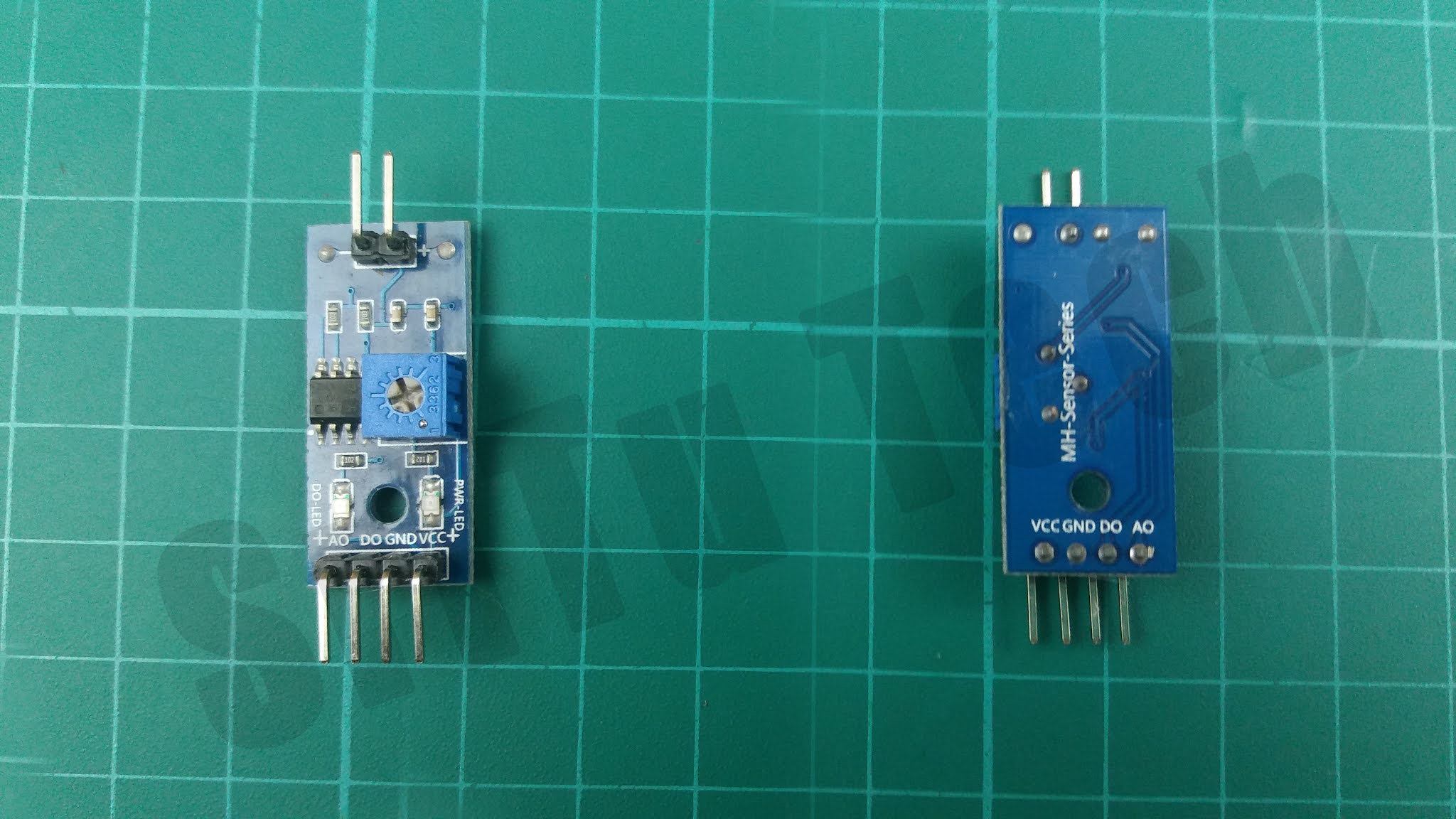This is designed to be attached to the Arduino board. For this, the module includes an analog PIN and a digital PIN. The main function of this module is to generate the output voltage according to the resistance value received through the probe. We can get it as a signal from Analog and Digital pins. Also, the Potentiometer here can change the sensitivity of the digital value. Also here we can see four pins. The work done by them is given below.
VCC     –         supply voltage (5v)
GND     –         The GND connection of the module
AO         –        Analog output. From this pin, we can get a value of 0 – 1024. We need to connect this                            PIN to an analog PIN on the Arduino board.
DO         –        Digital output. We can get a value of 1 or 0. We need to connect this PIN to a digital                                PIN on the Arduino board.
Let us now see how this sensor works.
The test part(Probe) of this sensor is designed to include two conductors. Also, these two conductors act as a variable resistor. This resistance is inversely proportional to the moisture content of the soil. As the amount of water in the soil increases, the conductivity increases and less resistance occurs. Also, as the amount of water in the soil decreases, the conductivity decreases and more resistance occurs. This resistor produces an output voltage and we can measure the moisture by measuring it. That task is performed by the module.
Well, now we will test how this sensor works using Arduino. The necessary accessories are given below. You can easily buy these components using the links below.
Okay, we will learn this project step by step.

### Step 1

First, identify these components.

Arduino Nano boardYou can use any other Arduino board for this project.
Soil moisture sensor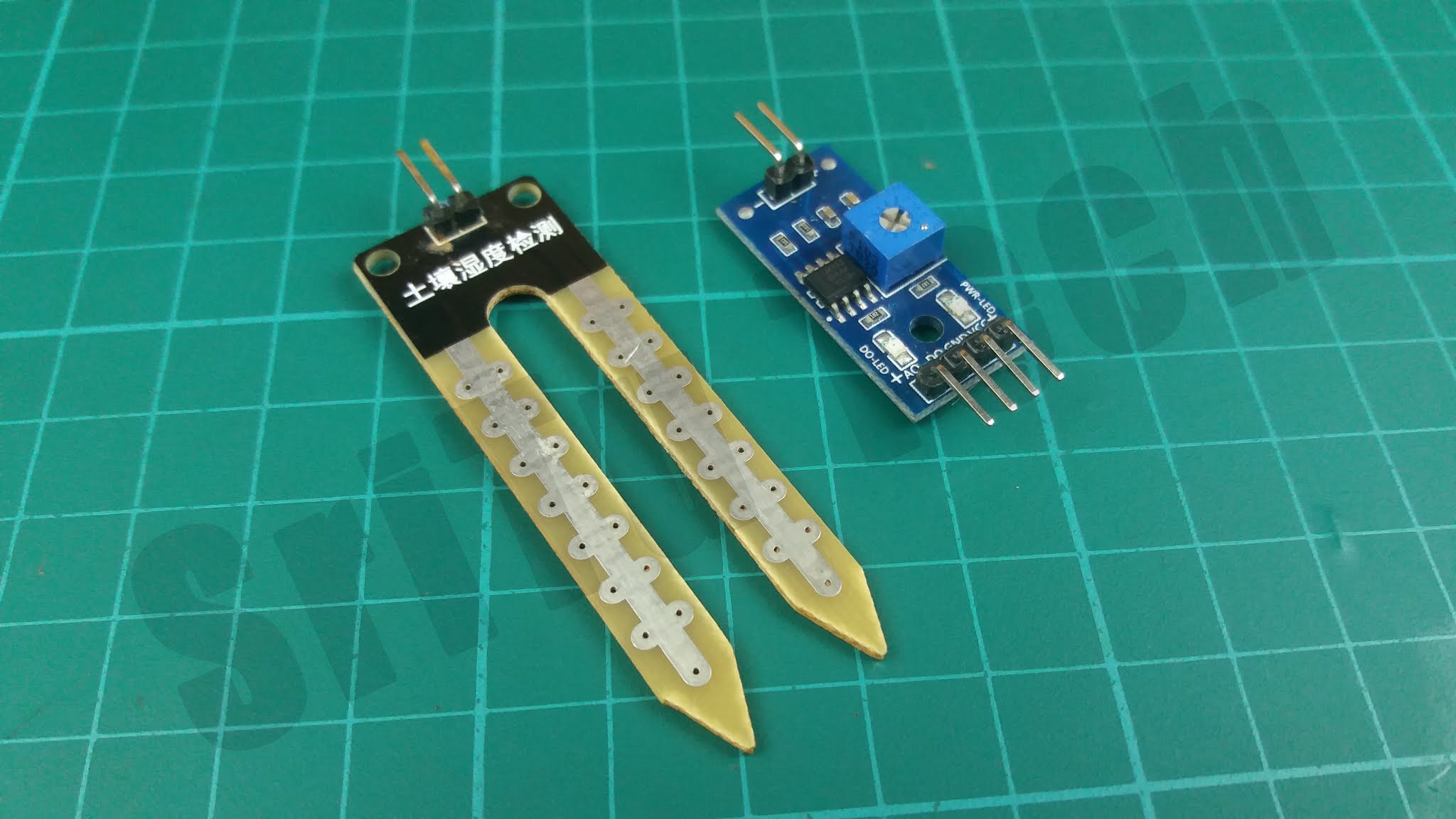Described aboveYou can use this to easily connect components.

Jumper wires

###<br /> (adsbygoogle = window.adsbygoogle || []).push({});<br /> Step 2

Now we can connect these components. For that, use the following circuit diagram.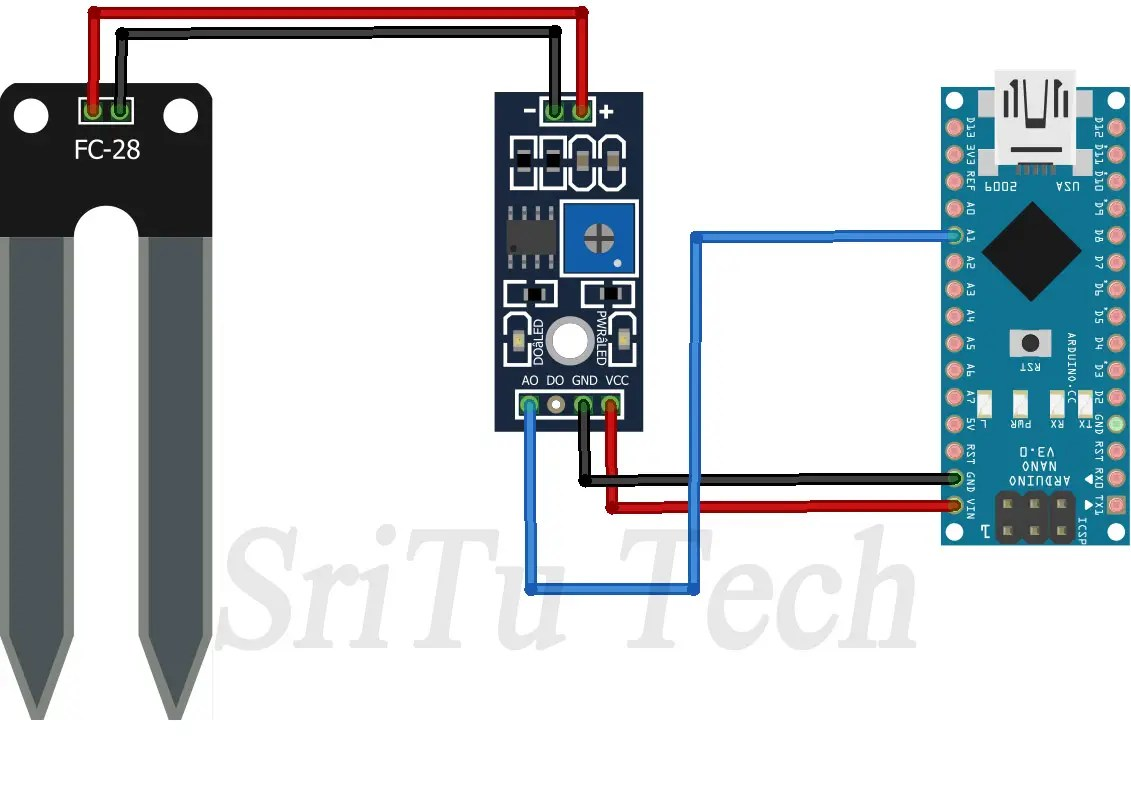Now we have to create the required program for this project. The required program is given below.
The complete program of this project – Download
``````/*How to work soil hydrometer moisture sensor.
created by the SriTu Tech team.
Read the code below and use it for any of your creation
*/

void setup() {
Serial.begin(9600);//enable serial monitor
}

void loop() {
Serial.print("Moisture value : ");//print text
Serial.println(value);//print value
}``````

#### Code explanation

The serial monitor is enabled in void setup first.
void setup() {
Serial.begin(9600);//enable serial monitor
}

Then read the sensor value in the Void loop and put it in the integer variable. Finally, that value is displayed in the serial monitor.
void loop() {
Serial.print(“Moisture value : “);//print text
Serial.println(value);//print value

### Step 4

Now we have to upload this program to the Arduino board. For that select the correct board and port and upload the program.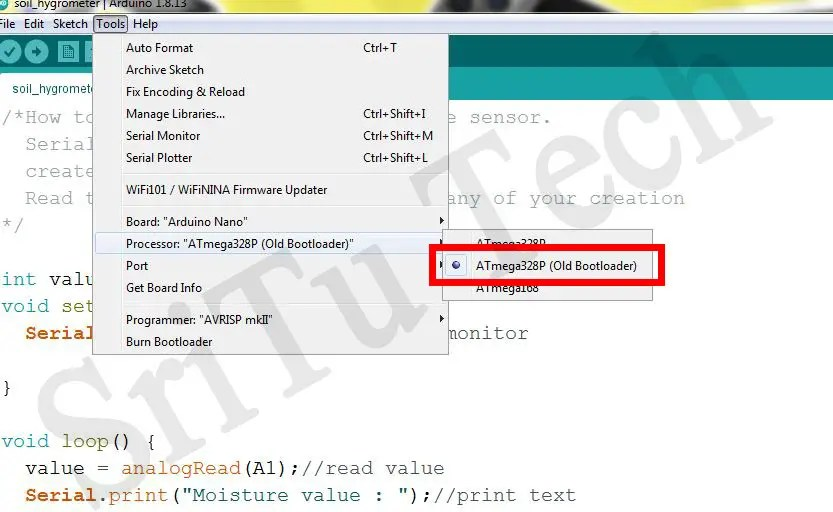OK, enjoy this tutorial. The full video guide is given below. So, we will meet in the next tutorial.